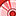Announcements
The way to achieve your own success is the willingness to help somebody else. Go for it!
cancel
Showing results for
Did you mean:Contributor III

## Year Over Year Growth Calculation

Hello,

I am having difficulty trying to calculate YOY growth for my average and penetration metrics, for example, average sales. The Sales, Units, Sales PY, Units PY, Avg. Sales, and Avg. Sales PY calculate fine. The formula for them are below:

Sales = Sum(sales)

Units = Sum(units)

Sales PY = Sum({<Year={\$(=only(Year)-1)}>}sales)

Units PY = Sum({<Year={\$(=only(Year)-1)}>}units)

Avg. Sales = Sum(sales) / Sum(units)

Avg. Sales PY = Sum({<Year={\$(=only(Year)-1)}>}sales) / Sum({<Year={\$(=only(Year)-1)}>}units)

However, my Avg. Sales Growth formula does not work as expected. The formula is below:

Avg. Sales Growth = ((Sum(sales)/Sum(units)) -
(Sum({<Year={\$(=only(Year)-1)}>}sales) / Sum({<Year={\$(=only(Year)-1)}>}units))) /
(Sum({<Year={\$(=only(Year)-1)}>}sales) / Sum({<Year={\$(=only(Year)-1)}>}units))

If Avg. Sales for the current year is \$3.80 and Avg. Sales PY is \$3.41, Avg. Sales Growth is being calculated as 11.6%. But it should be 11.4%... how is this happening and how do I resolve? Thanks!

Labels (1)
• ### Qlik Sense

3 RepliesMVP

Only(Year) is something not working in your case due to there is nothing aggregate to your field where you compare. Perhaps this may will give hint

((Sum(sales)/Sum(units)) -
(Sum({<Year={\$(=Max(Year-1))}>}sales) / Sum({<Year={\$(=Max(Year-1))}>}units))) /
(Sum({<Year={\$(=Max(Year-1))}>}sales) / Sum({<Year={\$(=Max(Year-1))}>}units))

Before develop something, think If placed (The Right information | To the right people | At the Right time | In the Right place | With the Right context)Specialist

hi,

try this

((Sum({<Year={\$(=only(Year)-1)}>}sales) / Sum({<Year={\$(=only(Year)-1)}>}units)/Sum({<Year={\$(=only(Year))}>}sales) / Sum({<Year={\$(=only(Year))}>}units))*100)-100.

ksrinivasanContributor III
Author

Hi everyone,

Just an update to this that the rounding of dollar and percent value is what is causing the discrepancy. If the full number is used in the YOY calculation, it is correct.Tags
Community Browser